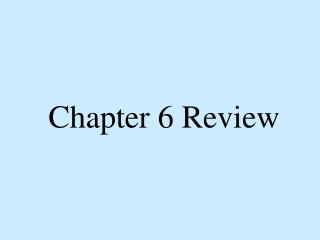# Chapter 6 Review - PowerPoint PPT PresentationDownload PresentationChapter 6 Review

Chapter 6 Review
Download Presentation## Chapter 6 Review

- - - - - - - - - - - - - - - - - - - - - - - - - - - E N D - - - - - - - - - - - - - - - - - - - - - - - - - - -
##### Presentation Transcript

1. Chapter 6 Review

2. Name the net • A. square prism • B. square pyramid • C. triangular prism • D. triangular pyramid

3. Name the net • A. cone • B. circular pyramid • C. cylinder • D. triangular prism

4. Name the net • A. square prism • B. square pyramid • C. triangular prism • D. triangular pyramid

5. Name the net • A. square prism • B. square pyramid • C. triangular prism • D. triangular pyramid

6. Name the net • A. square prism • B. square pyramid • C. triangular prism • D. triangular pyramid

7. Name the net • A. cone • B. cylinder • C. triangular prism • D. triangular pyramid

8. Name the geometric solid • A. rectangular prism • B. square pyramid • C. triangular prism • D. triangular pyramid

9. Name the geometric solid • A. rectangular prism • B. rectangular pyramid • C. triangular prism • D. triangular pyramid

10. Name the geometric solid • A. circular pyramid • B. square pyramid • C. cylinder • D. cone

11. Name the geometric solid • A. cone • B. square pyramid • C. cylinder • D. triangular pyramid

12. Name the geometric solid • A. square prism • B. square pyramid • C. triangular prism • D. triangular pyramid

13. Name the geometric solid • A. square prism • B. square pyramid • C. triangular prism • D. triangular pyramid

14. Find the perimeter of the shape shown below. • A. 14 ft • B. 40 ft • C. 24 ft. • D. 28 ft 4 ft 10 ft

15. Find the circumferenceof the circle shown. • A. 31.4 • B. 15.7 • C. 78.5 • D. 3.14 5 in

16. Find the area of the parallelogram 7 cm • A. 15 • B. 56 • C. 30 • D. 112 8cm

17. Find the perimeter of the trapezoid 8 in • A. 140 • B. 110 • C. 132 • D. 45 14 in 11 in 12 in

18. Find the area of the circle 6 in. • A. 18.84 • B. 28.26 • C. 37.68 • D. 11,304

19. Find the area ofthe trapezoid 5 mm 7mm 6mm • A. 45.5 • B. 120 • C. 26 • D. 39 8 mm

20. Find the perimeter of the triangle 16 yds 10 yds • A. 60 • B. 38 • C. 160 • D. 120 12 yds

21. Find the area of the rectangle 20 ft • A. 160 • B. 80 • C. 140 • D. 1200 60 ft

22. Estimate the area of the shape. • A. 7 • B. 10 • C. 14 • D. 19 6

23. A square table has an area of81 square feet. What is the length? • A. 8 • B. 9 • C. 10 • D. 11

24. A large square billboard has an area of 400 square yards. How long is the billboard? • A. 40 • B. 100 • C. 20 • D. 80

25. The square root of 56 is closest to which whole number? • A. 6 • B. 7 • C. 8 • D. 9

26. The square root of 90 is closest to which whole number? • A. 8 • B. 9 • C. 10 • D. 11